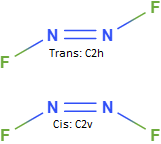# Difluorodiazine (FNNF) may exist as both the cis- and trans- isomers. The Raman and IR...

## Question:

Difluorodiazine (FNNF) may exist as both the cis- and trans- isomers.

The Raman and IR vibrational spectra of a pure sample of one of these isomers show the bands listed below and no other bands are observed: IR 989,421,360 cm^-1 and Raman 1636,1010, 592 cm^-1.

a. Draw Lewis structures for the two isomers and give the point group.

b. For each isomer calculate the number and symmetry of normal modes of vibration and identify the modes that are IR or Raman active. Show your work.

c. Indicate whether the vibrational modes are IR or Raman active. Does your answer agree with the observed spectra? Deduce which isomer the pure sample is.

d. Explain how you can distinguish between these isomers without calculating the symmetry of vibrational modes.

## Determining the Vibrational Modes of a Compound:

Every compound is able to translate, rotate, and vibrate in space. Each of these motions can be characterized by an irreducible representation. Translational and rotational motion is easy to determine as these motions align with the cartesian and rotational axes (which are typically noted in a character table); the process for determining the vibrational modes is somewhat involved and will be demonstrated below using the cis-difluorodiazine molecule.

To begin, determine the point group of the compound and obtain the character table; the cis-isomer belongs to the {eq}C_{2v} {/eq} point group and the character table is seen below:

{eq}\begin{array}{|C|C|C|C|C|C|C|} \hline C_{2v} & E & C_{2(z)} & \sigma_{v(xz)} & \sigma_{v(yz)} & Linear & Quadratic\\ \hline A_{1} & 1 & 1 & 1 & 1 & z & x^{2} + y^{2}, z^{2} \\ \hline A_{2} & 1 & 1 & -1 & -1 & R_{z} & xy \\ \hline B_{1} & 1 & -1 & 1 & -1 & x, R_{y} & xz \\ \hline B_{2} & 1 & -1 & -1 & 1 & y, R_{x} & yz \\ \hline \end{array} {/eq}

To determine the irreducible representations corresponding to the vibrations of this compound, we must extract them by first generating the total reducible representation of this molecule and then excluding the translational and rotational modes. This is done in 3 steps:

1. Multiply the sum of the translational modes (labeled below as "XYZ") by the number of atoms unchanged by each operation (labeled "Unmoved")
2. Subtract the translational ("XYZ") and rotational ("R(xyz)" modes from this product
3. Decompose this difference ("Vibrations") into its fundamental irreducible representations.

These steps are tabulated below:

{eq}\begin{array}{|C|C|C|C|C|C|C|} \hline C_{2v} & E & C_{2(z)} & \sigma_{v(xz)} & \sigma_{v(yz)}\\ \hline XYZ & 3 & -1 & 1 & 1 \\ \hline Unmoved & 4 & 0 & 4 & 0 \\ \hline \Gamma & 12 & 0 & 4 & 0 \\ \hline XYZ & 3 & -1 & 1 & 1 \\ \hline R(xyz) & 3 & -1 & -1 & -1 \\ \hline Vibrations & 6 & 2 & 4 & 0 \\ \hline \end{array} {/eq}

This reducible representation ("Vibrations") can be decomposed by inspection to: {eq}3A_{1},A_{2},2B_{1} {/eq}

For (a), the trans isomer belongs to the {eq}C_{2h} {/eq} point group as the only non-identity operations that apply to it are a {eq}C_{2} {/eq} rotation, a horizontal mirror operation, and (naturally) an inversion through the central point. The cis isomer also has a {eq}C_{2} {/eq} rotational axis, but has instead two vertical mirrors and no inversion center; it belongs to the {eq}C_{2v} {/eq} point group.For (b), 6 vibrational modes were calculated and determined for the cis isomer in the above section with an expected 5 IR bands and 6 Raman bands. The same process has been repeated for the trans isomer below.

{eq}\begin{array}{|C|C|C|C|C|C|C|} \hline C_{2h} & E & C_{2(z)} & i & \sigma_{h}\\ \hline XYZ & 3 & -1 & -3 & 1 \\ \hline Unmoved & 4 & 0 & 0 & 4 \\ \hline \Gamma & 12 & 0 & 0 & 4 \\ \hline XYZ & 3 & -1 & -3 & 1 \\ \hline R(xyz) & 3 & -1 & 3 & -1 \\ \hline Vibrations & 6 & 2 & 0 & 4 \\ \hline \end{array} {/eq}

This decomposes to: {eq}3A_{g},A_{u},2B_{u} {/eq}

The trans isomer would have the following active bands:{eq}A_{u}, 2B_{u} {/eq} would be IR active and {eq}3A_{g} {/eq} would be Raman active.

For (c),the cis isomer would have the following active bands: {eq}3A_{1} {/eq} and {eq}2B_{1} {/eq} are both IR and Raman active, while {eq}A_{2} {/eq} is only Raman active.

The trans isomer would have the following active bands:{eq}A_{u}, 2B_{u} {/eq} would be IR active and {eq}3A_{g} {/eq} would be Raman active.

The compound is undoubtedly the trans isomer.

For (d), the cis isomer belongs to the {eq}C_{2v} {/eq} point group, where every irreducible representation is Raman active. As both molecules necessarily have 6 vibrational modes, this would then require the cis isomer to have 6 Raman active bands. As the same did not have so many Raman active bands (and indeed had 6 vibrational modes with no overlap between Raman and IR active bands), it undoubtedly proved the compound to be the trans isomer.